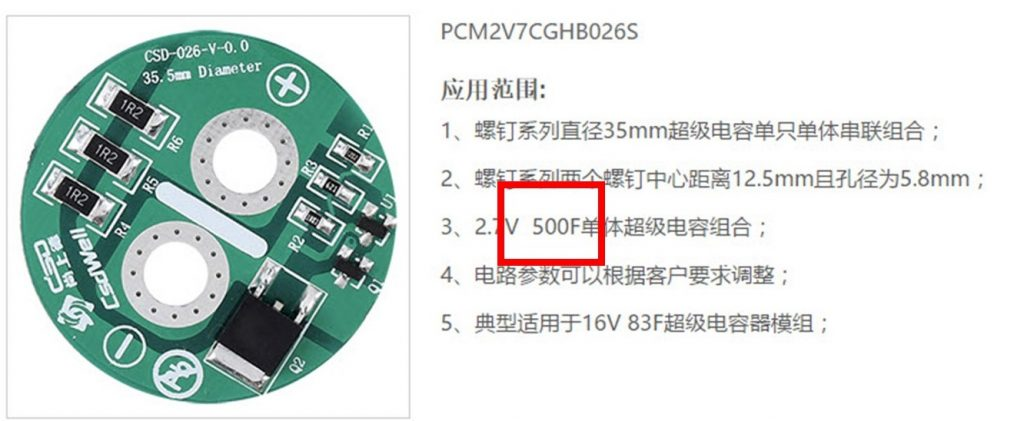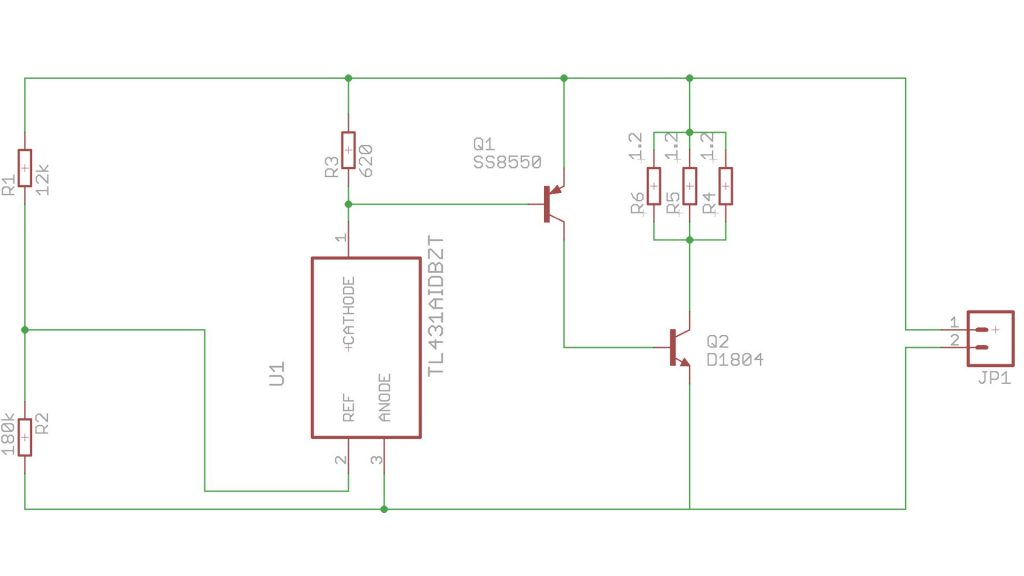## Simple and cheap way to protect your super caps (video #139 )

In video #133 we used super capacitors to safely shutdown the Rapberry Pi. In the comments, viewers asked about over voltage protection of the super caps. I will show you a big and small and cheap possibility. And I will show you one concept which does not really work.

But why do we have to protect Super Capacitors? And how can we protect them? Most Super capacitors are only specified up to 2.7 volt. If we need higher voltages, we have to connect a few of them in series. To give the Raspberry a save shutdown I used two caps because it runs on 5 volt USB.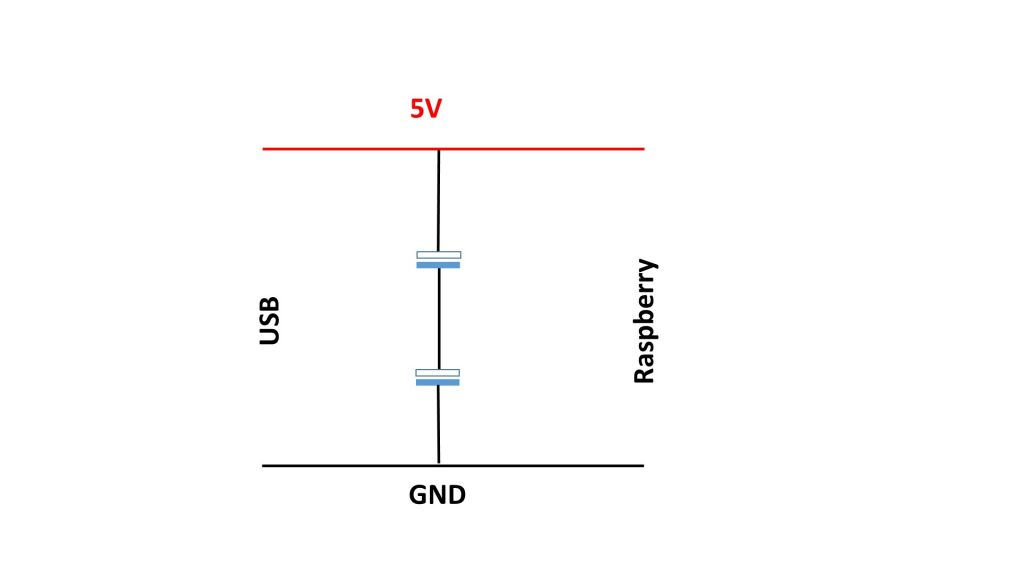If we charge the capacitors, the same current I flows through each of the caps. If one cap has a smaller capacitance, it charges faster and its voltage can go above the maximum 2.7 volts. To prevent this, we can connect a resistor and a switch in parallel to the capacitors. If the switch is closed, part of the current flows through the resistor and the voltage stays below the 2.7 volts.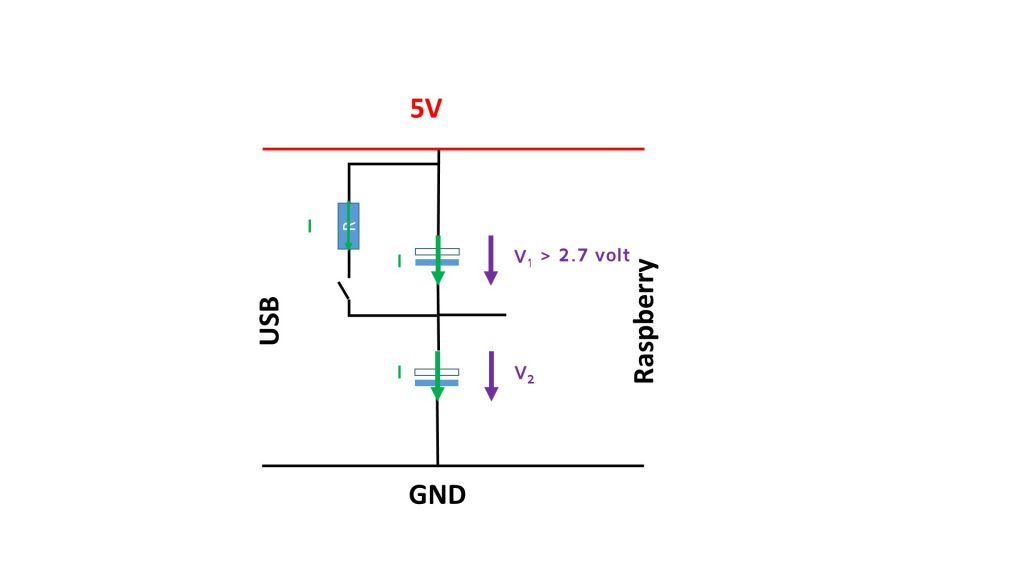We also can replace the resistor and the switch by a 2.4 volts Zener diode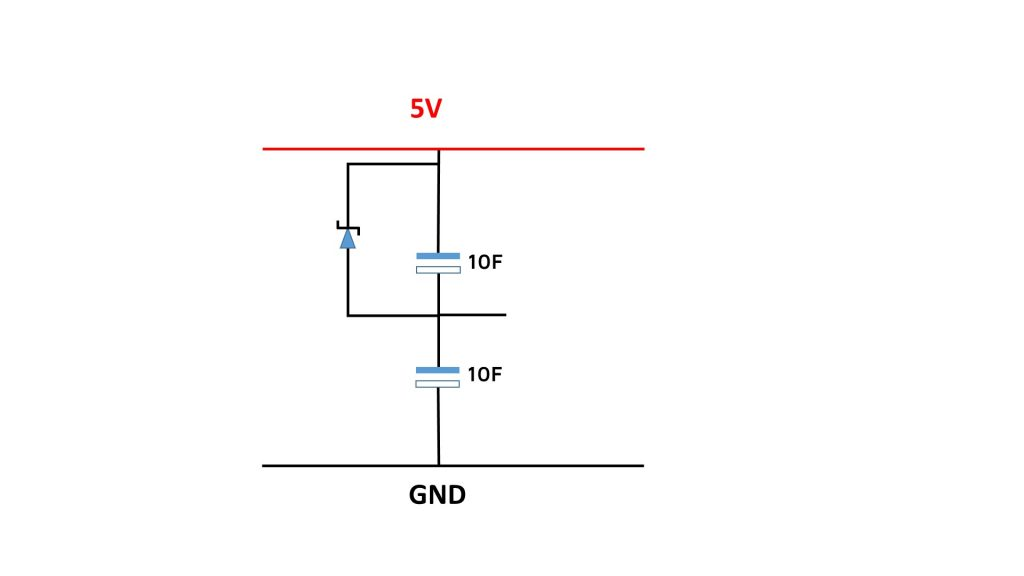This is a solution for small currents. A better and cheaper solution is to replace the Zener diode with a TL431. This part behaves like a Zener diode with a variable Zener voltage. So, the final diagram looks like that: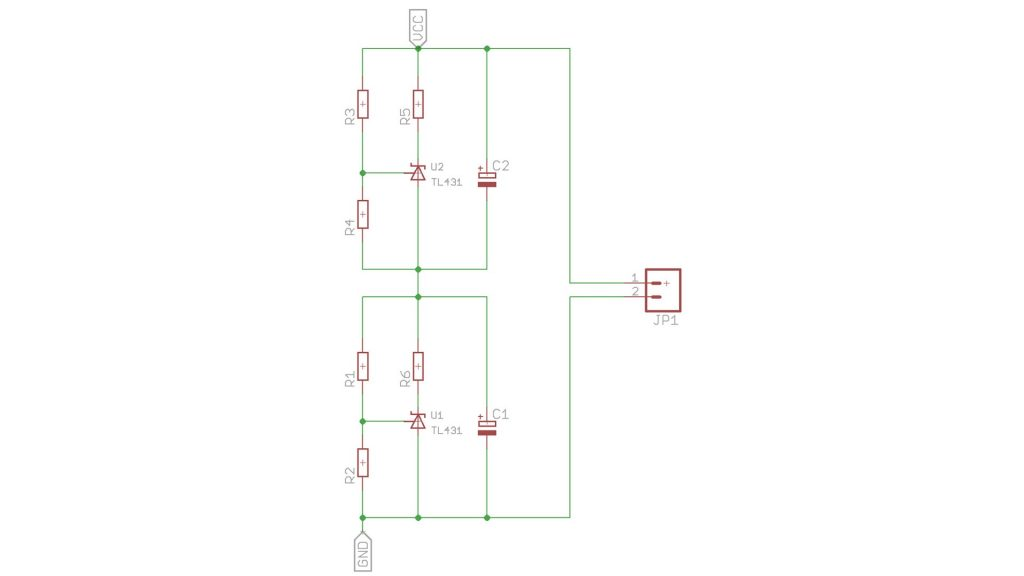A TL431 can handle up to 150 mA

The formula for the TL431 is: V=(1+R1/R2) x 2.5

And for the resistors: (R4=R2 and R3=R1): R2=0.08 x R1